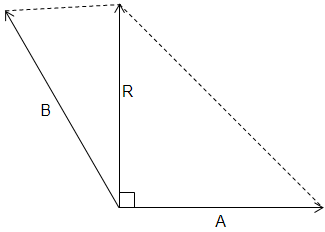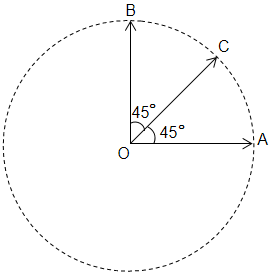A blind person after walking 10 steps in one direction, each of length 80 cm, turns randomly to the left or to the right by $90°.$ After walking a total of 40 steps the maximum possible displacement of the person from his starting position could be -

(A)

(B)

(C)

(D)

Concept Questions :-

Resultant of Vectors
High Yielding Test Series + Question Bank - NEET 2020

Difficulty Level:

The resultant of two vectors A and B is perpendicular to the vector A and its magnitude is equal to half the magnitude of vector B. The angle between A and B is -1. $120°$

2.  $150°$

3.  $135°$

4.  None of these

Concept Questions :-

Resultant of Vectors
High Yielding Test Series + Question Bank - NEET 2020

Difficulty Level:

The three vectors OA, OB and OC have the same magnitude R. Then the sum of these vectors have magnitude -(A)  $\mathrm{R}$

(B)  $\sqrt{2}\mathrm{R}$

(C)  $3\mathrm{R}$

(D)  $\left(1+\sqrt{2}\right)\mathrm{R}$

Concept Questions :-

Resultant of Vectors
High Yielding Test Series + Question Bank - NEET 2020

Difficulty Level:

A truck travelling due north with 20m/s turns towards west and travels at the same speed. Then the change in velocity is -

(A)  40 m/s north-west

(B)  $20\sqrt{2}$ m/s north-west

(C)  40 m/s south-west

(D)  $20\sqrt{2}$ m/s south-west

High Yielding Test Series + Question Bank - NEET 2020

Difficulty Level:

A particle is moving in a circle of radius r centered at O with constant speed v. The change in velocity moving from A to B $\left(\angle \mathrm{AOB}=40°\right)$ is -

(A)

(B)

(C)

(D)

Concept Questions :-

Resultant of Vectors
High Yielding Test Series + Question Bank - NEET 2020

Difficulty Level:

If $\stackrel{\to }{A}=\stackrel{\to }{B}+\stackrel{\to }{C}$ and magnitudes of A, B and C are 5, 4 and 3 units respectively, the angle between A and C is -

(A)

(B)

(C)

(D)  $\mathrm{\pi }/2$

Concept Questions :-

Resultant of Vectors
High Yielding Test Series + Question Bank - NEET 2020

Difficulty Level:

What is the resultant of three coplanar forces:  at $0°$ at $30°$ and  at $150°$?

1.

2.

3.

4.

Concept Questions :-

Resultant of Vectors
High Yielding Test Series + Question Bank - NEET 2020

Difficulty Level:

Two forces, ${\mathrm{F}}_{1}$ and ${\mathrm{F}}_{2}$ are acting on a body. One force is doubled of the other force and the resultant is equal to the greater force. Then the angle between the two forces is -

(A)

(B)

(C)

(D)

Concept Questions :-

Resultant of Vectors
High Yielding Test Series + Question Bank - NEET 2020

Difficulty Level:

If the angle between vector a and b is an acute angle, then the difference a$-$b is -

(A)  the main diagonal of the parallelogram

(B)  the minor diagonal of the parallelogram

(C)  any of the above

(D)  none of the above

Concept Questions :-

Resultant of Vectors
High Yielding Test Series + Question Bank - NEET 2020

Difficulty Level:

What displacement must be added to the displacement   to give a displacement of  pointing in the x-direction?

(A)

(B)

(C)

(D)

Concept Questions :-

Resultant of Vectors